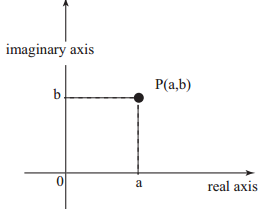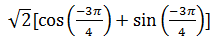# Argand Plane and Polar representation of Complex Number

## Argand Plane

Complex number z=a+ib can be represented by a point P(a,b) on a xy plane. The x -axis represent the real part while the y-axis represent the imgainary part
Such a diagram is called Argand Plane or Complex planePoints to Note
(1) The length OP is called the module of z and is denoted by |z|
|z|=$\sqrt{(x^2+y^2)}$
OP=$\sqrt{(x^2+y^2)}$
(2) Purely real number lies on X-axis while purely imaginary number lies on y-axis
(3) The Line OP makes an angle $\theta$ with the positive direction of x-axis in anti-clockwise sense is called the argument or amplitude of z
It is given by
$\theta=tan^{-1}\frac{y}{x}$
(4) Argument of a complex number is not unique since if $\theta$ is the value of argument then $2n\pi + \theta$ (n=0, $\pm$1, $\pm$2, ....) are also values of the argument. Thus, argument of complex number can have infinite number of values which differ from each other by any multiple of $2\pi$
(5) The unique value of $\theta$ such that $\pi < \theta <= \pi$ is called the principal value of the amplitude or principal argument
(6) The principal argument of the complex number is find using the below steps
Step 1) for z=a+ib , find the acute angle value of $\theta=tan^{-1}|\frac{y}{x}|$
Step 2) Look for the values of a ,b
if (a,b) lies in First quadrant then Argument=$\theta$
if (a,b) lies in second quadrant then Argument =$\pi-\theta$
if (a,b) lies in third quadrant then Argument =$-\pi+\theta$
if (a,b) lies in Fourth quadrant then Argument =$-\theta$

(7) The conjugate of the complex number lies symmetrically about the x-axis
(8) All the real number can be represent as complex number with zero imaginary part. like a +i(0) . So we can term real numbers as subset of bigger set of complex numbers

## Vector Representation of the complex number

Just like a vector,A complex number on the argand plane for two things modulus and arg(z) which is direction. So all the complex number can be defined as position vector on the argand plane.
The addition ,substraction of complex number can happen just like vector addition and substraction

## Polar Representation of the complex number

We can represent complex number is terms of polar coordinates
Let r be any non negative number and $\theta$ any real number. If we take $x=rcos\theta$ and $y=rsin\theta$ then, $r=\sqrt{x^2+y^2}$which is the modulus of z and $\theta=tan^{-1}\frac{y}{x}$ which is the argument or amplitude of z and is denoted by arg.z
we also have $x+iy=r(cos\theta+isin\theta)=r[cos(2n\pi+\theta)+isin(2n\pi+\theta)]$ , where n=0, $\pm$1, $\pm$2, ....
The unique value of $\theta$ such that $\pi < \theta <= \pi$ is called the principal value of the amplitude or principal argument
So we will be writing the polar coordinates form of complex number in principal argument form
How to convert any complex number in Polar form
Step 1) for z=a+ib , find the acute angle value of $\theta=tan^{-1}|\frac{y}{x}|$
Step 2) Look for the values of a ,b
if (a,b) lies in First quadrant then Argument=$\theta$
if (a,b) lies in second quadrant then Argument =$\pi-\theta$
if (a,b) lies in third quadrant then Argument =$-\pi+\theta$
if (a,b) lies in Fourth quadrant then Argument =$-\theta$
Step 3) Polar form = |z|[cos (arg) + isin (arg)]

Examples:
1. Find the modulus and amplitude for the complex number
z=-1-i
Solution
We have already given the steps for modulus and arg
Modulus
|z|=$\sqrt{(x^2+y^2)}$
How to find the arg
Step 1) for z=a+ib , find the acute angle value of $\theta=tan^{-1}|\frac{y}{x}|$
Step 2) Look for the values of a ,b
if (a,b) lies in First quadrant then Argument=$\theta$
if (a,b) lies in second quadrant then Argument =$\pi-\theta$
if (a,b) lies in third quadrant then Argument =$-\pi+\theta$
if (a,b) lies in Fourth quadrant then Argument =$-\theta$
So
|z|=$\sqrt{2}$
Acute angle
$\theta=tan^{-1}|\frac{y}{x}|$
$\theta=\frac{\pi}{4}$
Now the complex lies in third quadrant
So $arg=\frac{-3\pi}{4}$
2) Find the polar coordinate equation for the above complex number
Solution we know the polar coordinate equation is given by
Polar form = |z|[cos (arg) + i sin (arg)]
so
\$z=